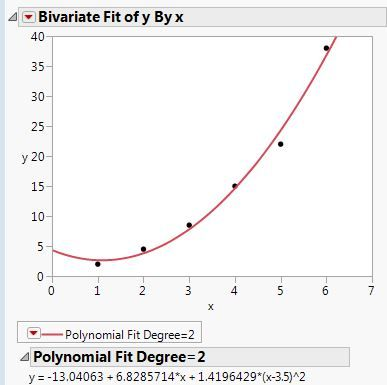Our World Statistics Day conversations have been a great reminder of how much statistics can inform our lives. Do you have an example of how statistics has made a difference in your life? Share your story with the Community!
Choose Language Hide Translation Bar
Highlighted

## Form of best fit polynomials

I have a pretty basic question that I cannot find the solution to....

When performing a polynomial fit as shown below...Is there a way to get the resulting polynomial in the typical form like that below?

y = c0 + c1*x + c2*x^2

Im sure there is a clever reason for giving fit results in the JMP default form...please enlighten me as to why.

Thx,

Rory

1 ACCEPTED SOLUTION

Accepted Solutions
Highlighted

## Re: Form of best fit polynomials

JMP automatically centers variables when higher order terms are added to the model. This practice is recommended to reduce the collinearity among predictors to, in turn, reduce the variance inflation. (x^2 is highly collinear with x otherwise.)

There are two ways to get the form that you want:

1. Fit Using the Uncentered Variable: Click the red triangle next to Bivariate Fit and select Fit Special. The option to center variables is near the bottom. Here you can also select the second-order polynomial model to be fit.
2. Convert the Model Afterward: Save the equation as the prediction formula. Open the formula, click the red triangle and select Simplify.

Hope one of these ways is satisfactory.

Learn it once, use it forever!
4 REPLIES 4
Highlighted

## Re: Form of best fit polynomials

JMP automatically centers variables when higher order terms are added to the model. This practice is recommended to reduce the collinearity among predictors to, in turn, reduce the variance inflation. (x^2 is highly collinear with x otherwise.)

There are two ways to get the form that you want:

1. Fit Using the Uncentered Variable: Click the red triangle next to Bivariate Fit and select Fit Special. The option to center variables is near the bottom. Here you can also select the second-order polynomial model to be fit.
2. Convert the Model Afterward: Save the equation as the prediction formula. Open the formula, click the red triangle and select Simplify.

Hope one of these ways is satisfactory.

Learn it once, use it forever!
Highlighted

## Re: Form of best fit polynomials

Hi Mark,

Thanks for your explanation. Is there any reference (e.g. paper, book) that we can use to justify the centering practice for higher order terms? Look forward to your recommendation.

Claire

Highlighted

## Re: Form of best fit polynomials

The practice of centering regressor variables is covered and recommended in practically all textbooks about regression analysis. Many softwares do not provide this option or if they do, it is not the default, however. JMP decided to make it the default many years ago when there are higher order terms in the linear model. It makes no difference when all the terms are first order.

Here is a commonly cited source specifically about regression problems, including collinearity:

• Belsley, D. A., Kuh, E., and Welsch, R. E. (1980). Regression Diagnostics: Identifying Influential Data and Sources of Collinearity. New York: John Wiley & Sons.

Learn it once, use it forever!
Highlighted
Article Labels

There are no labels assigned to this post.